A car going initially with a velocity 13.5 m/s accelerates at a rate of 1.9 m/s 2 for 6.2 s. It then accelerates at a rate of -1.2 m/s2 unti

Question

A car going initially with a velocity 13.5 m/s accelerates at a rate of 1.9 m/s 2 for 6.2 s. It then accelerates at a rate of -1.2 m/s2 until it stops. a) Find the car’s maximum speed b) Find the total time from the start of the first acceleration until the car is stopped c) What’s the total distance the car travels?

in progress 0
6 months 2021-07-28T14:40:45+00:00 1 Answers 2 views 0

1. (a) For the first 6.2 s, the car has velocity at time t given by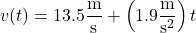so that after 6.2 s, it attains a velocity of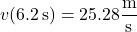For any time t after 6.2 s, its velocity is given by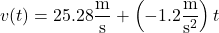which tells us the velocity only falls from this point onward. This means the maximum speed is 25.28 m/s, or about 25.3 m/s.

(b) Solve for t (after 6.2 s) that makes v(t) = 0 :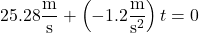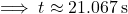It takes the car about 21.2 s to come to a rest, so the car travels a total of about 6.2 s + 21.2 s = 27.4 s.

(c) For the first 6.2 s, the car undergoes a displacement at time t of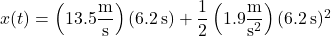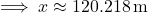For time t beyond 6.2 s, its displacement is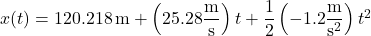The car comes to a rest after 21.2 s (accelerating at a rate of -1.2 m/s^2), so that its total displacement is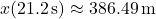so the car travels a total distance of about 387 m.